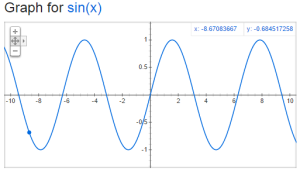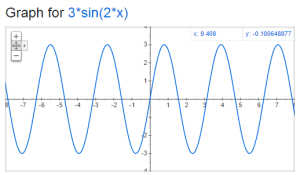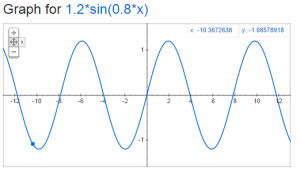# 1.07 Sinusoidal signals

The next few sections in The Arts of Electronics deals with signals.  What are the characteristics of signals?  Amplitude, frequency, shape, etc.

The values we are looking at is voltage as a function of time.  The most basic signal is the sinusoidal signal.  Have you ever graphed y=sin(x) and end up with:But how would you compare this wave to the following:There are two key things to think about when comparing sine waves:  frequency and amplitude.    The amplitude is the distance from y=0 to the top of the sine wave.  The frequency is how many times the wave repeats in a given period of time.  We typically use the unit hertz (Hz) for frequency, which is one cycle (or period) per second.  Lets take a look at period and amplitude in our original sine graph:The formula that you should remember is

V=A*sin(2*pi*f*t)

A is the amplitude in volts, f is the frequency in Hz and t is the time in seconds.